Numerical Method for One-Dimensional Convection-diffusion EquationUsing Radical Basis Functions | OMICS International
Journal of Physical Mathematics
All submissions of the EM system will be redirected to Online Manuscript Submission System. Authors are requested to submit articles directly to Online Manuscript Submission System of respective journal.

# Numerical Method for One-Dimensional Convection-diffusion EquationUsing Radical Basis Functions

Su LD1,2*, Jiang ZW2 and Jiang TS2

1North-Eastern Federal University, Belinskogo, Yakutsk, Russia

2Department of Mathematics, Linyi University, Linyi, P.R. China

*Corresponding Author:
Ling-De Su
Department of Mathematics, North-Eastern
Federal University, Belinskogo, Yakutsk, Russia
Tel: +79676245017
E-mail: [email protected]

Received Date: February 10, 2015; Accepted Date: April 29, 2015; Published Date: May 08, 2015

Citation: Su LD, Jiang ZW, Jiang TS (2015) Numerical Method for One-Dimensional Convection-diffusion Equation Using Radical Basis Functions. J Phys Math 6:136. doi:10.4172/2090-0902.1000136

Copyright: © 2015 Su LD, et al. This is an open-access article distributed under the terms of the Creative Commons Attribution License, which permits unrestricted use, distribution, and reproduction in any medium, provided the original author and source are credited.

Visit for more related articles at Journal of Physical Mathematics

#### Abstract

In this paper, the meshless method is employed for the numerical solution of the one-dimensional (1D)
convection-diffusion equation based on radical basis functions (RBFs). Coupled with the time discretization and the collocation method, the proposed method is a truly meshless method which requires neither domain nor boundary discretization. The algorithm is very simple so it is very easy to implement. The results of numerical experiments are presented, and are compared with analytical solutions to confirm the good accuracy of the presented scheme

#### Keywords

Meshless method; Radical basis function (RBF); Numerical solution; Convection-diffusion equation

#### Introduction

Whenever we consider mass transport of a dissolved species (solute species) or a component in a gas mixture, concentration gradients will cause diffusion. If there is bulk fluid motion, convection will also contribute to the flux of chemical species. Therefore, we are often interested in solving for the combined effect of both convection and diffusion.

The convection-diffusion equation is a combination of the diffusion and convection (advection) equations, and describes physical phenomena where particles, energy, or other physical quantities are transferred inside a physical system due to two processes: diffusion and convection .

The general convection-diffusion equation has the following form [2,3]:

In the above equation, four terms represents transient, convection, diffusion and source term respectively. Where u(x, t) is the variable of interest (species concentration for mass transfer, temperature for heat transfer), D is the diffusivity (also called diffusion coefficient), ν is the average velocity that the quantity is moving, R(x, t) is source term represents capacity of internal sources, ∇ represents gradient and ∇. represents divergence.

This paper is devoted to the numerical computation of the onedimension

(1D) convection-diffusion equation: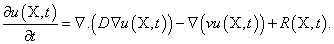With the initial conditions: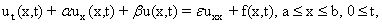(1.1)

With the initial conditions: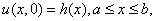(1.2)

And Dirichlet boundary conditions: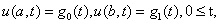(1.3)

Where α, β and ε are known constant coefficients, h(x) and gi (t) (i =0,1) are known continuous functions.

Recently, much attention has been given to the development, analysis, and implementation of stable methods for the numerical solution of the convection-diffusion equations (see  and the reference therein). Jim Douglas, et al.  combine definite element and finite difference methods based on the method of characteristic for solving the convection-diffusion problems. Chen and Hon  consider the 2D and 3D Helmholtz and convection-diffusion equation using boundary knot method. The meshless local Petro-Galerkin method for convection-diffusion equation was considered in . A new finite difference method described by Ram P. Manohar and John W. Stephenson .

In this article, we present a numerical scheme to solve the convection-diffusion equation using the collocation method with Radial Basis Function (RBF). The results of numerical experiments are presented, and are compared with analytical solutions to confirm the good accuracy of the presented scheme.

In last 25 years, the radial basis functions (RBFs) method is known as a powerful tool for scattered data interpolation problem. The use of RBFs as a meshless procedure for numerical solution of partial differential equations is based on the collocation scheme. Because of the collection technique, this method does not need to evaluate any integral. The main advantage of numerical procedures which use RBFs over traditional techniques is mesh-less property of these methods. RBFs are used actively for solving partial differential equations. The examples see [9-11]. In the last decade, the development of the RBFs as a truly meshless method for approximating the solutions of PDEs has drawn the attention of many researchers in science and engineering [12-14]. Meshless method has become an important numerical computation method, and there are many academic monographs are published [15-17].

The layout of the article is as follows: In section 2, we introduce the collocation method and apply this method on the convection-diffusion equation. The results of numerical experiments are presented in section 3. Section 4 is dedicated to a brief conclusion. Finally, some references are introduced at the end.

#### The Collocation Method with Radical Basis Function

The approximation of a distribution u(x), using RBF may be written as a linear combination of N radial functions, usually it takes the following form: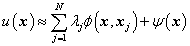, for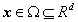(2.1)

Where N is the number of data points, x=(x1, x2…xd), d is the dimension of the problem, the λ’s are coefficients to be determined and φ is the radial basis function. Eq. (2.1.1) can be written without the polynomial ψ. In that case, φ must be unconditional positive definite to guarantee the solvability of the resulting system (e. g. Gaussian or Inverse Multi quadrics). However, ψ is usually required when φ is conditionally positive definite, i. e, when φ has a polynomial growth towards infinity. We will use the Multi quadrics (MQ), which defined as: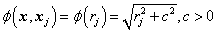(2.2)

Where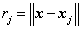is the Euclidean norm. Since φ given by (2.2) is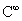continuous, we can use it directly.

If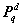denotes the space of d-variate polynomial of order not exceeding than q, and letting the polynomials (P1,P2, …, Pm) be the basis ofin Rd, then the polynomial ψ(x) in Eq. (2.1) is usually written in the following form: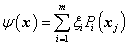(2.3)

Where m = (q-1+d)!/(d!(q-1)!). To get the coefficients x=(λ1, λ2… λN), and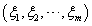the collocation method is used. However, in addition to the N equations resulting from collecting Eq. (2.1.1) at N points and extra m equations are required. This is ensured by the m conditions for Eq. (2.1),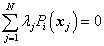(2.4)

In a similar representation as Eq. (2.1), for any linear partial differential operator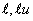u can be approximated by: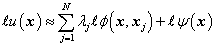(2.5)

The convection-diffusion equation

Let us consider the 1D convection-diffusion equation Eq. (1.1), with the initial conditions Eq. (1.2) and the Dirichlet boundary conditions Eq. (1.3).

First, let us discretize Eq. (1.1) according to the following θ-weighted scheme: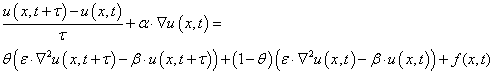(2.6)

where 0 ≤θ ≤1 , and τ is the time step size, and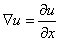using the notation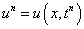where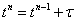, we get: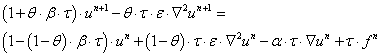(2.7)

Assuming that there are N − 2 interpolation points,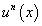can be approximated by: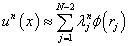,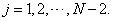(2.8)

To guarantee the positive definition, here we use the following approximation: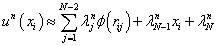(2.9)

Whereis the Euclidean norm. The additional conditions due to Eq. (2.4) are written as: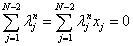(2.10)

Writing Eq. (2.9) together with Eq. (2.10) in a matrix form we have: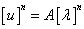(2.11)

Where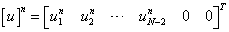,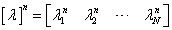and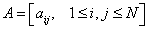is given by: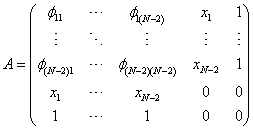(2.12)

Assuming that there are p < N − 2 internal points and N −2 − p boundary points, then the N ×N matrix A can be split into: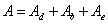, where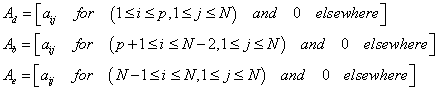(2.13)

Using the notation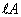to designate the matrix of the same dimension as A and containing the elements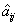where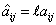, 1≤ i, j ≤ N , then Eq. (2.2.1) together with the boundary conditions Eq. (1.3) can be written in matrix form as: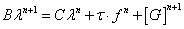(2.14)

where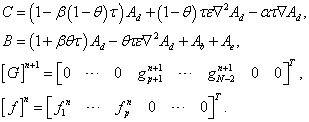(2.15)

Eq. (2.14) is obtained by combining Eq. (2.6), which applies to the domain points, while Eq. (1.3) applies to the boundary points. Together with the initial condition Eq. (1.2) and Eq. (2.14), we can get all λ’s, thus we can get the numerical solutions.

Since the coefficient matrix is unchanged in time steps, we use the LU factorization to the coefficient matrix only once and use this factorization in our algorithm.

Remark: Although Eq. (2.14) is valid for any value of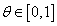, we will use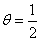(The famous Crank-Nicolson scheme).

#### Numerical Examples

In this section, we present several numerical results to confirm the efficiency of our algorithm for solving the 1D convection-diffusion equation.

Example 1

In this example, we consider the convection-diffusion Eq. (1.1) in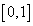with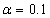,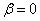,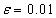, with the boundary conditions: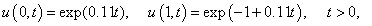And the initial condition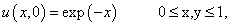Then the analytical solution of the equation is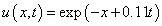. The right side functions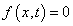.

We use MQ radical basis function for the computation, the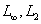and RMS errors and Root-Mean-Square (RMS) of errors are obtained in Table 1 for t = 0.1, 0.3, 0.5, 0.7 and 1.0 with time steps τ = 0.001 and dx = 0.001 .

T 0.1 0.3 0.5 0.7 1.0
L -errors 1.547×10-5 2.371×10-4 3.599×10-4 4.639×10-4 5.909×10-4
L2 -errors 6.788×105 1.787×10-4 3.453×10-4 3.453×10-4 4.372×10-4
RMS- errors 6.778×10-5 1.784×10-4 3.448×10-4 3.448×10-4 4.365×10-4

Table 1: For T=0.1, 0.3, 0.5, 0.7 and 1.0 with time steps T=0.001 and dx=0.001.

The space-time graph of analytical and numerical solutions for t=1 are given in Figure 1. Note that we cannot distinguish the exact solution from the estimated solution in Figure 1.

Figure 1: Space-time graph of the exact and estimated solutions with T=1 for Example 1.

Example 2

In this example, we consider Eq. (1.1) with α =1 , β = 0 , ε =1 and the boundary conditions: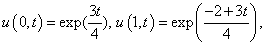And the analytical solution of the equation is given as: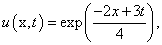We get the initial conditions from the exact solution. The right side functionsTheand RMS errors and Root-Mean-Square (RMS) of errors are obtained in Table 2 for T=0.1, 0.25, 0.5, 0.75 and 1.0 with time steps τ = 0.001 and dx 0.01 .

T 0.1 0.25 0.5 0.75 1.0
L -errors 8.855×10-5 1.031×10-4 1.245×10-4 1.501×10-4 1.811×10-4
L2 -errors 5.929×10-5 6.882×10-5 8.305×10-5 1.002×10-4 1.208×10-4
RMS- errors 5.842×10-5 6.781×10-5 8.183×10-5 9.870×10-5 1.191×10-4

Table 2: Numerical Errors at different times with T=0.001 and dx=0.001 for Example 2.

Similar to the previous example, the space-time graph of analytical and estimated solutions for t=1 are presented in Figure 2.

Figure 2: The space-time graph of exact and numerical solutions with T=1 for Example 2.

Example 3

We consider the convection-diffusion equation Eq. (1.1) with α = −1 , β =10 and ε =1 in the interval, the exact solution is given as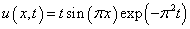. The boundary conditions are: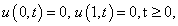The right side functions of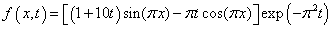, and we extract the initial conditions from the exact solution.

These results are obtained with dx = 0.001 , τ = 0.001 . Similar to the previous examples, the L and L2 error and RMS errors for t =0.5, 0.75, 1.0, 1.25 and 1.5 are presented in Table 3.

T 0.1 0.25 0.5 0.75 1.0
L -errors 1.547×10-5 2.112×10-6 2.472×10-7 2.673×10-8 2.757×10-9
L2 -errors 1.179×10-5 1.595×10-6 1.859×10-7 2.007×10-8 2.066×10-9
RMS- errors 1.177×10-5 1.593×10-6 1.856×10-7 2.104×10-8 2.063×10-9

Table 3: Numerical Errors at different times with T=0.001 and dx=0.001 for Example 3.

The space-time graph of analytical and numerical solution for t=1 is presented in Figure 3.

Example 4

In this example, we consider the convection-diffusion equation in [0,1] has the following form: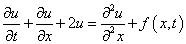The right side functions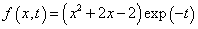, with the boundary condition: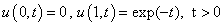Then the analytical solution of the equation is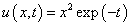we get the initial conditions from the exact solution.

In this case, we use the radial basis functions MQ for the discussed scheme. These results are obtained for dx = 0.001 , τ = 0.001. The graph of analytical and numerical solution for t=0.1, 0.2, 0.3, 0.4 and 0.5 is given in Figure 4. The results obtained show the very good accuracy and efficiency of the new approximate scheme. Note that we cannot distinguish the exact solution from the estimated solution in Figure 4.

We also give the difference between exact solutions and numerical solutions in Figure 5.

#### Conclusion

In this paper, the collocation method is employed for the numerical solution of convection-diffusion equation based on radical basis functions (RBFs). Coupled with the time discretization, the proposed method is a truly meshless method which requires neither domain nor boundary discretization. The results of numerical experiments are presented, and are compared with analytical solutions confirmed the good accuracy of the presented scheme.

#### Acknowledgements

This work was supported by the national Natural Science Foundation of China (Grant Nos.11301252, 11201212) and Applied Mathematics Enhancement Program of Linyi University.

#### References

Select your language of interest to view the total content in your interested language

### Article Usage

• Total views: 14985
• [From(publication date):
November-2015 - Nov 20, 2019]
• Breakdown by view type
• HTML page views : 10829Can't read the image? click here to refresh